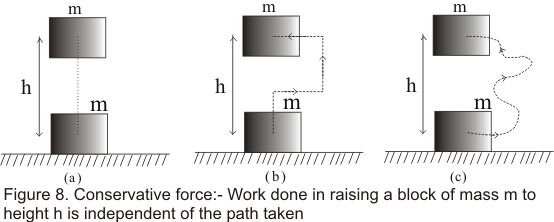# Conservative Forces

## (11) Conservative Forces

• Consider the gravitational force acting on a body .If we try to move this body upwards by applying a force on it then work is done against gravitation
• Consider a block of mass m being raised to height h vertically upwards as shown in fig 8(a) .Work done in this case is mgh
• Now we make the block travel the path given in figure 8(b) to raise its height h above the ground.In this path work done during the horizontal motion is zero because there is no change in height of the body due to which there would be no change in gravitational PE of the body and if there is no change in the speed KE would also remains same
• Thus for fig 8(b) if we add up the work done in two vertical paths the result we get is equal to mgh• Again if we move the the block to height h above the floor through an arbitrary path as shown in fig 8(c) ,the work done can be calculated by breaking the path into elementary horizontal and vertical portions
• Now work done along the horizontal path would be zero and along the vertical paths its add up to mgh
• Thus we can say that work done in raising on object against gravity is independent of the path taken and depends only on the initial and final position of the object
• Now we are in position to define the conservative forces
" If the work done on particle by a force is independent of how particle moves and depends only on initial and final position of the objects then such a force is called conservative force"
Gravitational force,electrostatic force ,elastic force and magnetic forces are conservative forces
• Total work done by the conservative force is zero when particle moves around any closed path returning to its initial position
• Frictional forces and viscous forces are examples of non-conservative forces as these forces always oppose the motion and result in loss in KE
• Concept of Potential Energy is associated with conservative forces only .No such PE is associated with non-conservative forces like frictional forces
• Mathematically Potential Energy function U is defined if Force F can be written as
$F_s= \frac {dU}{ds}$
Where F_s is the component of force in the direction of ds
or
dU = -F_s.ds
• For One-dimensional motion, this can be written as
$F= -\frac {dU}{dx}$
or
dU= -F.dx
or
$U_A - U_B = \int_{x_a}^{x_b} fdx$
• For three dimensional motion,this can be written as
$\mathbf{F}=-\frac{\partial U}{\partial x}\mathbf{i} -\frac{\partial U}{\partial y}\mathbf{j} -\frac{\partial U}{\partial z}\mathbf{k}$

Watch this tutorial for more information on How to solve work-energy problem